Select Page

# CBSE Maths 12 Science Application of Derivatives Answers for MCQ in English

CBSE Maths 12 Science Application of Derivatives Answers for MCQ in English to enable students to get Answers in a narrative video format for the specific question.

Expert Teacher provides CBSE Application of Derivatives Maths 12 Science Answers for MCQ through Video Answers in English language. This video solution will be useful for students to understand how to write an answer in exam in order to score more marks. This teacher uses a narrative style for a question from Application of Derivatives not only to explain the proper method of answering question, but deriving right answer too.

Please find the question below and view the Answer in a narrative video format.

Question:

## Similar Questions from CBSE, 12th Science, Maths, Application of Derivatives

Question 1 : What is the maximum value of the function sin x+ cos x? (View Answer Video)

Question 2 : The line y = x + 1 is a tangent to the curve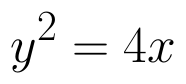at the point: (View Answer Video)

Question 3 : It is given that  at x=1, the function attains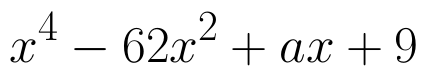its maximum value on the interval[0,2]. Find the value of a? (View Answer Video)

Question 4 : The function f is a differentiable function and satisfies the functional equation f(x) + f(y) = f(x + y) – xy – 1for every pair x, y of real numbers. If f(1) = 1, then the number of integers n ≠ 1 for which f(n) = n is_______________. (View Answer Video)

Question 5 : Find the maximum value of in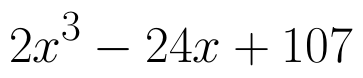the interval [1,3]. find the maximum value of the same function in [-3,-1]. (View Answer Video)

### Inverse Trigonometric Functions

Question 1 : Write the principal value of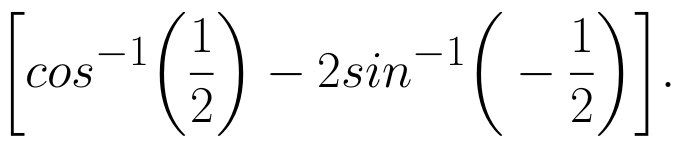(View Answer Video)

Question 2 : Solve for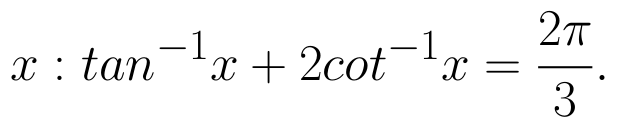(View Answer Video)

Question 3 :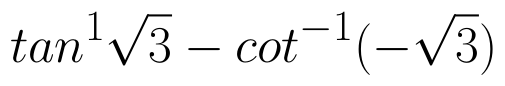is equal to :

Question 4 : Write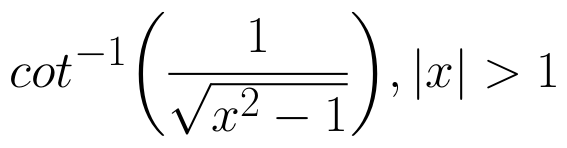in the simplest form. (View Answer Video)

Question 5 : If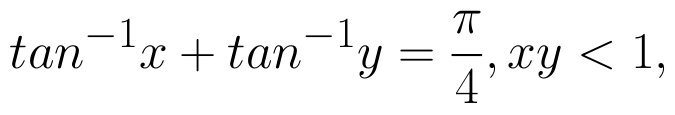then write the value of x + y + xy. (View Answer Video)

### Linear Programming

Question 1 : The objective function is maximum or minimum, which lies on the boundary of the feasible region. (View Answer Video)

### Vector Algebra

Question 1 :  P and Q are two points with position vectors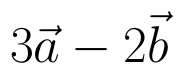and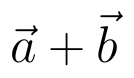respectively. Write the position vector of a point R which divides the line segment PQ externally in the ratio 2:1.  (View Answer Video)

Question 2 : Compute the magnitude of the following vectors: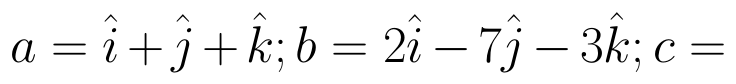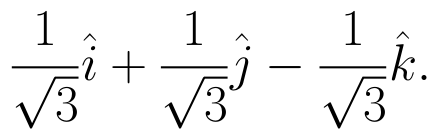(View Answer Video)

Question 3 : Find the scalar quantities from the following:
(i) Time period          (ii) Distance          (iii) Force
Question 5 : Find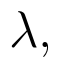if the vectors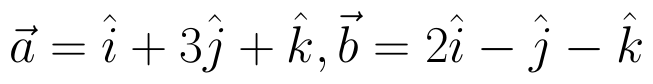and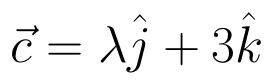are coplanar.   (View Answer Video)